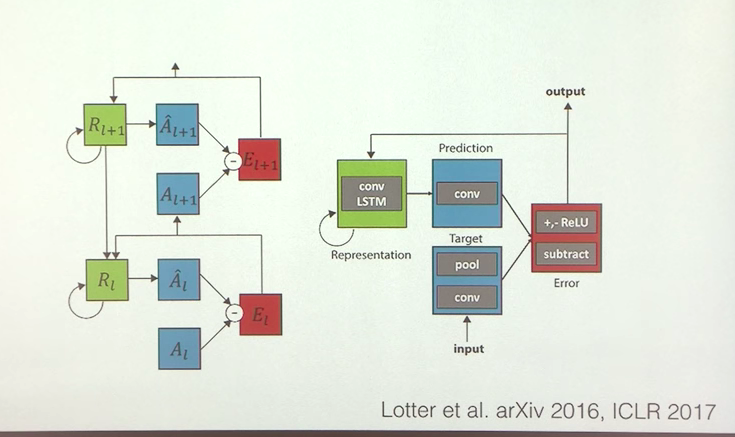# Predictions As Perception Notes

## Notes from a Youtube video Predictive Coding Models of Perception By David Cox from IBM

The researchers developed a neural network called PredNet. It is trained on videos and its objective is: Given a few frames of a video, predict the next frame. This process involves minimizing the error between the predicted frame and the actual frame. Only the error in prediction is passed to the next layer. The next layer's objective is to predict the error and then try to minimize it, and so on. This concept is directly inspired from the theory of Predictive Coding. They show evidence that a network trained this way also learns very interesting concepts (Like predicting the angle of rotation for a face etc.). Here is a diagram of the network they used:Some notes on the following diagram:

• Errors propagate forward. Each layer receives the input, and then should produce a prediction on the input, then calculate the difference between the input and the prediction. This difference is the error.
• The main task of the network is to minimize errors in prediction.